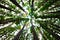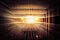# Introduction to Constraints, Assertions, and Triggers

When you create a database, it usually with a few core goals in mind: to store data in an easy manner, and to be able to easily access or manipulate it. For the latter, this could for example be to insert new data into the database or delete useless and outdated data. However, one of the challenges that arise when we want to modify our database — that we may violate the integrity constraints of the database. To avoid violating these constraints, it is possible to use multiple methods. …

# An Introduction to Hash Tables

In this article, we will consider the data structure, Hash Tables. To do so, we will first understand the different parts of it and its structure. We will consider both linear and extensible Hash Tables. Afterward, we will see how we can perform different operations on them, i.e., searching, insertion, and deletion. In the end, we will wrap it up with an explanation of the pros and cons of using the different Hash Tables to store and access data.

# Understanding the Hash Table

A Hash Table is a data structure, where we store the data in an associative manner. …

# Introduction to B-treesPhoto by Arnaud Mesureur on Unsplash

One of the most used data structures in computer science is the tree. This has resulted in many different versions being developed. In this article, we will become better acquaintances with the so-called B-trees. To do so, we will first understand the different parts of it and its structure. Afterward, we will see how we can perform different operations on them, i.e., searching, insertion, and deletion. In the end, we will wrap it up with an explanation of the pros and cons of using B-trees to store data.

# Understanding the B-tree

The B-tree is a so-called balanced tree, meaning that all paths from…

# Introduction to Data IntegrationPhoto by Joshua Sortino on Unsplash

In this article, we will talk about the concept of data integration. It is a concept that is needed, when we consider data and how it is stored. Usually, data is stored in databases with a specific structure and norm for how it is inserted into the database. What happens, though, when we have multiple databases, and we want to merge them? This is where we need data integration. Simply put, data integration is the process of taking several databases and making the data in these sources work together as if they were one single database.

# Too Many Databases Spoil the Data

Before we dive more…

# The Basics of Syntax Analysis: Context-Free Grammar

In our former series of articles, we looked at the basics of lexical analysis. In this article, we will start the series concerning syntax analysis. In the first article concerning lexical analysis, we said that we can identify tokens/patterns with the help of regular expressions and pattern rules. There is, though, a limit to lexical analysis — while we can look at the individual tokens, but it cannot check the syntax of a given sentence. Therefore, we need syntax analysis. We will start by giving both an informal and formal definition of context-free grammar.

Defining Context-Free Grammar

Imagine that you…

# The Basics of Lexical Analysis: From Regex to DFA

In the former articles, we have been introduced to both Regular Expressions and Finite Automata’s. We have also seen how we can convert an NFA to its DFA equivalent. In this article, we will combine the knowledge we have obtained from all the former articles, and we will try to represent Regular Expressions as a DFA. We will start by showing how some very simple Regular Expressions can be shown as an NFA. Then we can use these examples as building blocks as we move on to more advanced examples. …

# Discrete Math — The Basics of Lexical Analysis: From NFA to DFA

In the former two articles, we have been introduced to both Regular Expressions and Finite Automata’s. In this article, we will try to understand how we can convert an NFA to a DFA. This will be used in the next article, where will see how we can express Regular Expressions through a DFA — but we will need to know how to convert from an NFA to a DFA. So, let us start.

## The Idea Behind

It is possible to say that every DFA is an NFA, but not every NFA is a DFA. However, every NFA has a DFA equivalent. That means…

# Discrete Math — The Basics of Lexical Analysis: DFA and NFA

In the former article, we talked about one of the complete basic concepts in Lexical Analysis: Regular Expressions. In this article, we will expand our knowledge of Lexical Analysis and talk about Finite Automata — both Non-Deterministic (NFA) and Deterministic (DFA). We need to understand these concepts and in the next article, we will explore how we can convert an NFA to a DFA.

The Concept of Finite Automata

Let us first understand the concept of a Finite Automata itself before we continue. A Finite Automata is basically an abstract machine — it is the simplest machine to recognize patterns…

# Discrete Math — The Basics of Lexical Analysis: Regex

In this article, we will talk about one of the most basic subjects in Lexical Analysis: Regular Expressions, which are also called Regex. This will create the foundation for the coming articles, where we will explore what Non-Deterministic and Deterministic Finite Automata’s are — and how they can be used to represent Regular Expression. But first — we will need to define what is meant by Regex.

## Regular Expressions

The question is — what is a regular expression and what is it used for? Regular Expressions, also called Regex, are extremely effective in extracting information from a text. In other words, Regular…

# Graph Theory — Finding The Shortest Paths

In former articles, we looked at various kinds of graphs and algorithms defined upon them. We defined the two algorithms: Prim’s and Kruskal’s algorithm. They are both used for the same purpose — finding the minimum spanning tree of a weighted graph but offer two different methods. In this article, we will look at Dijkstra’s Algorithm. It is used to find all the shortest paths from the chosen root to all vertices in the given graph. We will see how the algorithm works in this article and compare it to the two former methods. …## Naja Møgeltoft

Data science and Machine Learning student at Copenhagen University.